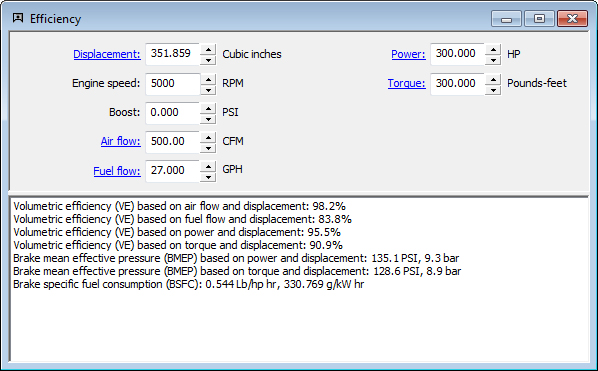## Efficiency Calculation

The efficiency calculation calculates volumetric efficiency (VE), brake mean effective pressure (BMEP), and brake specific fuel consumption (BSFC). Support is provided for turbocharger or supercharger boost.VE is calculated four different ways: by air flow, fuel flow, power, and torque. You can use any of these calculations you prefer. If they don't provide approximately the same result, you will know that something isn't right.

Similarly, BMEP is calculated from both torque and horsepower.

Engine efficiency graph